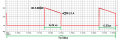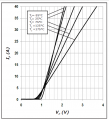# conduction power loss of SiC diode

#### orcuns91

Joined Nov 5, 2020
2
Hi everybody,
I'm trying to find the correct conduction loss value of a SiC Schottky diode. While the diode is in conduction, 33.5 A square wave current flows through it. I should use this current when determining the forward voltage in the forward characteristic graph in the datasheet, or is it 8.375 A, which is the average current value? You can also see the current waveform of the diode. (f=20 kHz, D=025) Thanks in advance.

#### Papabravo

Joined Feb 24, 2006
20,600
The forward voltage is determined at a particular current. Averages are meaningless in this context.

•orcuns91

#### Delta Prime

Joined Nov 15, 2019
1,311
Hello thereConduction losses are the average dissipated power in the diode during the forward
conduction phase.
Your on the right track.•orcuns91

#### Delta Prime

Joined Nov 15, 2019
1,311
To calculate maximum conduction losses, read maximum values of VF at IMin and IMax.
The first step is the calculation of the average and rms currents.
The forward average current is the output current: IF(AV) = Iload
The forward current has a trapezoidal shape." Please forgive me I don't know the
formula to calculate the rms current of trapezoidal waveform".•orcuns91

#### Papabravo

Joined Feb 24, 2006
20,600
To calculate maximum conduction losses, read maximum values of VF at IMin and IMax.
The first step is the calculation of the average and rms currents.
The forward average current is the output current: IF(AV) = Iload
The forward current has a trapezoidal shape." Please forgive me I don't know the
formula to calculate the rms current of trapezoidal waveform".1. Square the waveform
2. Take the average of the squared waveforn (≡ area under the curve)
3. Take square root of the average of the squared waveform
You can do it graphically as well

•orcuns91

#### Delta Prime

Joined Nov 15, 2019
1,311
@Papabravo. A thumbs up just isn't good enough. I salute you Sir. Thank you for the technical information.•orcuns91

#### crutschow

Joined Mar 14, 2008
33,342
Since the diode forward drop is relatively constant, (not ohmic) you use the average value of the current, not RMS to calculate the diode power loss.

•orcuns91

#### Papabravo

Joined Feb 24, 2006
20,600
Since the diode forward drop is relatively constant, (not ohmic) you use the average value of the current, not RMS to calculate the diode power loss.
I could not read the markings on the waveform scale. Does your average include the part where the diode is apparently(?) non-conducting?

•orcuns91

#### crutschow

Joined Mar 14, 2008
33,342
I could not read the markings on the waveform scale. Does your average include the part where the diode is apparently(?) non-conducting?
If the waveform is the current through the diode then there would be no non-conducting current.

•orcuns91

#### Papabravo

Joined Feb 24, 2006
20,600
If the waveform is the current through the diode then there would be no non-conducting current.
But the non-conducting (reverse(?)) current has nothing to do with the forward voltage.

•orcuns91

#### crutschow

Joined Mar 14, 2008
33,342
But the non-conducting (reverse(?)) current has nothing to do with the forward voltage.
Is that a significant value of current in this configuration?

•orcuns91

#### orcuns91

Joined Nov 5, 2020
2
Thank you for your answers. However, I still haven't been able to decide which Vf value to get. I increased the visibility of the current waveform and shared it in the attachment. Could you tell me what value should it get?#### Papabravo

Joined Feb 24, 2006
20,600
Is that a significant value of current in this configuration?
The graph of the current waveform is interesting in a pictorial sense, but I can't read any values, so I can't answer your question The original question revolved around a value for forward voltage when the diode was conducting. Which of the two values specified by the TS should be used, or is there another average value that you would prefer?

•orcuns91

#### Papabravo

Joined Feb 24, 2006
20,600
Thank you for your answers. However, I still haven't been able to decide which Vf value to get. I increased the visibility of the current waveform and shared it in the attachment. Could you tell me what value should it get?

View attachment 221528View attachment 221529
I would use (40 -27)/2 = 33.5 amperes as the average value of Vf.

•orcuns91

#### crutschow

Joined Mar 14, 2008
33,342
I would use (40 -27)/2 = 33.5 amperes as the average value of Vf.
I second the use of that value.

•orcuns91 and ericgibbs

#### ronsimpson

Joined Oct 7, 2019
2,795
Power loss: I am using the 75C line because the silicon in side the diode will be hot.
Should be using calculus to find the area under the curve of V*A.
Power loss for the on time =((40A @ 2.6V)+(27A @ 2.0V))/2 Approx.
Another option is to average the 40A and 27A and also average the 2.6 and 2.0V. Two watts less than above.

Next is to use duty cycle or on_off time.

•orcuns91

#### Delta Prime

Joined Nov 15, 2019
1,311
Last edited by a moderator:
•orcuns91